Scilab Home page | Wiki | Bug tracker | Forge | Mailing list archives | ATOMS | File exchange
Change language to: Français - Português - 日本語 - Русский

# cumsum

partial cumulative sums of the elements of an array

### Syntax

```y = cumsum(x)
y = cumsum(x, outtype)
y = cumsum(x, orientation)
y = cumsum(x, orientation, outtype)```

### Arguments

x

array of booleans, integers, real or complex numbers, polynomials, or rational fractions. Hypermatrices or sparse boolean or numerical matrices are supported as well.

orientation

This argument can be

• either a string with possible values `"*"`, `"r"`, `"c"` or `"m"`

• positive integer 1 ≤ orientation ≤ ndims(x): the index of the dimension along which the cumulative sum must be computed. 1 and "r", and 2 and "c", are equivalent.

outtype

Word `"native"` or `"double"`.

y

Array of size equal to that of `x`.

### Description

y = cumsum(x) computes and provides the partial cumulative sums `y(i)=sum(x(1:i))`, i.e.: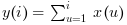y = cumsum(x, orientation) returns the partial cumulative sums of x along the dimension given by orientation:

• if orientation is equal to 1 or "r" then: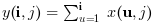, or for a N-Dimensional array:• if orientation is equal to 2 or "c" then: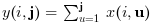, or for a N-Dimensional array: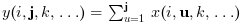• if orientation is equal to n then: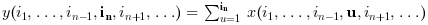• y = cumsum(x, "*") is equivalent to `y = cumsum(x)`

• y = cumsum(x, "m") is equivalent to `y = cumsum(x, orientation)` where orientation is the index of the first dimension of x that is greater than 1. This option is used for Matlab compatibility.

The outtype argument rules the way the summations are done:

• For arrays of floats, of polynomials, of rational fractions, the evaluation is always done using floating points computations. The `"double"` or `"native"` options are equivalent.

• For arrays of integers,

if `outtype="native"` the evaluation is done using integer computations (modulo 2^b, where b is the number of bits used),

if `outtype="double"` the evaluation is done using floating point computations.

The default value is `outtype="native"`.

• For arrays of booleans,

if `outtype="native"` the evaluation is done using boolean computations ( + is replaced with |),

if `outtype="double"` the evaluation is done using floating point computations (%t values are replaced by 1 and %f values by 0).

The default value is `outtype="double"`.When the input `x` is sparse, please keep in mind that the density of the result `y` will be almost always close to 100%.

### Examples

```A = [1,2;3,4];
cumsum(A)
cumsum(A,1)

I = uint8([2 95 103 ; 254 9 0])
cumsum(I) // native evaluation
cumsum(I,"double")
cumsum(I,2,"double")

s = poly(0,"s");
P = [s, %i+s ; s^2 , 1];
cumsum(P)
cumsum(P, 2)

B = [%t %t %f %f];
cumsum(B)          // evaluation in float
cumsum(B,"native") // similar to or(B)```